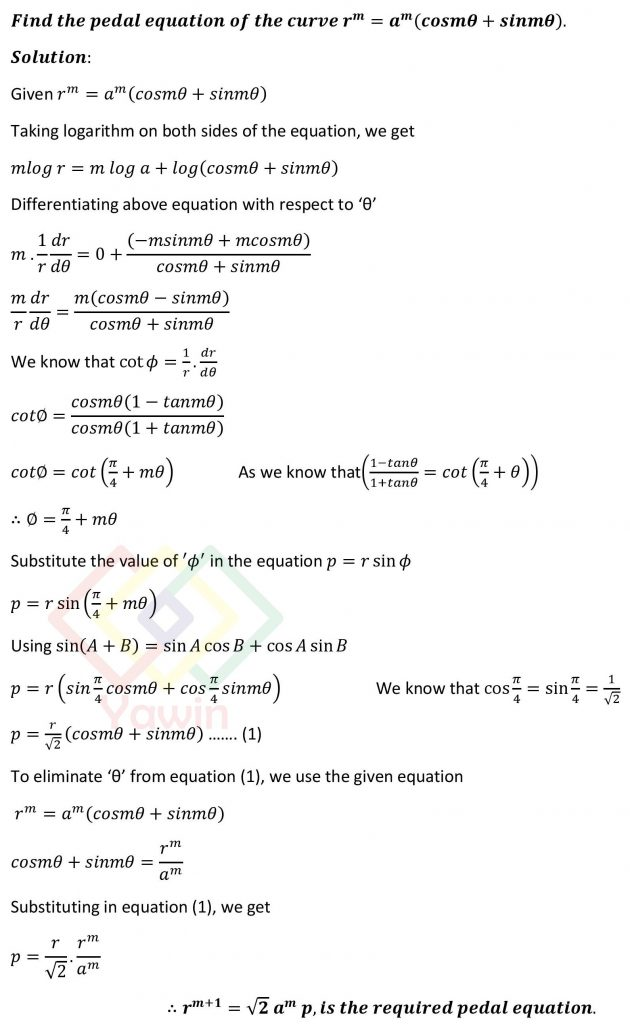Find the pedal equation of the curve r^m=a^m (cosm(theta)+sinm(theta)).

## Question## Question

Find the pedal equation of the curve r^m=a^m (cosm(theta)+sinm(theta)).

## Topic

Pedal equation of a polar curve